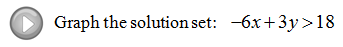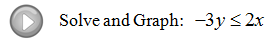## Algebra

Showing posts with label two variables. Show all posts
Showing posts with label two variables. Show all posts

### Systems of Linear Inequalities (Two Variables)

The systems of linear inequalities that we will be solving consist of two linear inequalities and two variables.  To solve these we will graph the solution sets of both linear inequalities and then determine where the sets intersect.  Any point in the overlap of the graphs will be a solution to the system.

Instructional Video: Systems of Linear Inequalities

Graph the solution set: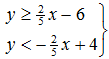The above solution suggests that (−5, 3) is a solution because it is shaded.  Check it and others to see if it solves both inequalities.These graphs can sometimes get messy so do your best to think about the solution before actually shading.  Use pencil and a good eraser when working on these problems.

Given the graph, determine the system.Graph the systems of inequalities.Graph the system: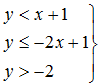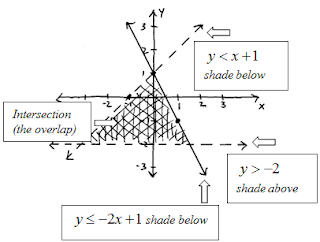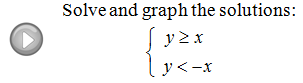### Linear Inequalities (Two Variables)

When graphing an equation like y = 3x − 6 we know that it will be a line.  The graph of a linear inequality such as y >= 3x − 6, on the other hand, gives us a region of ordered pair solutions.
Not only do the points on the line satisfy this linear inequality - so does any point in the region that we have shaded.  This line is the boundary that separates the plane into two halves - one containing all the solutions and one that does not. Therefore, from the above graph, both (0, 0) and (−2, 4) should solve the inequality.
Use a test point not on the boundary to determine which side of the line to shade when graphing solutions to a linear inequality.  Usually the origin is the easiest point to test as long as it is not a point on the boundary.

Graph the solution set.
If the test point yields a true statement shade the region that contains it.  If the test point yields a false statement shade the opposite side.

When graphing strict inequalities, inequalities without the equal, the points on the line will not satisfy the inequality; hence, we will use a dotted line to indicate this.  Otherwise, the steps are the same.

Graph the solution set.
Given the graph determine the missing inequality.